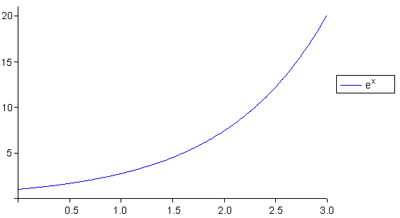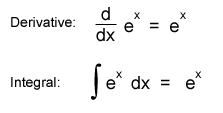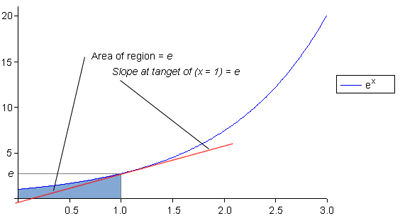# The Number e in Calculus

One of my closest math tutors once told me he had a student who asked the question: “Was math discovered or invented?”

I think the answer is probably ‘both’.  Some math I find beautiful.  Thinking about it can really clear my mind.  Especially the number ‘e’, which must have been discovered, since it has more to do with nature than with man.

Some Calculus Background:

In school math can get overly complicated. Maybe it is with good reason to challenge learners. For the most part I think it scares people away.  At this website calculus is not scary, but it is simplified. To tell you about the number ‘e’ and calculus, first I should explain that calculus is based on two concepts:

1. The first is finding the slope of a line tangent to a function. This is called a derivative.  Think of acceleration, which is the rate of change. As a rocket speeds up, it accelerates.
2. The second concept in calculus is finding the area under a function.   This is called an integral. Think of total distance traveled, or total fuel burned by a rocket.

These two concepts make up the fundamental theorem of calculus.  Intuitively, the acceleration of the rocket, the speed of the rocket, and the fuel it burns are related. Mathematically we use calculus to model the relationship.  It turns out it is possible to solve for each of the functions and tell the acceleration of the rocket and the total fuel burned – at any point in its trajectory!  The other amazing thing is, derivatives and integrals are inverse operations. This is sort of like multiplying and dividing.  It goes a little further though because we are dealing with rates of change. Instead of cutting apples into pieces, or multiplying rabbits, calculus allows us to look at relationships between units of measure as their rates change.

Consider a letter that is also a number: e = 2.718281828459045235…

When graphed [f(x) = ex], it looks like this:The number ‘e’ is important because it is tied to compounding growth. If a bank compounded interest daily instead of monthly, then hourly, then every minute, then every second, then every millisecond, and so on, mathematicians have found ‘e’ is arrived at. It represents the maximum performance level of any compounding activity. This applies to interest in the bank, reproducing bacteria, or the growing number of Napster shares in 2000.

Where calculus fits in: The function ex is it’s own derivate and integral.This tells us, at all points along the curve f(x) = ex, the slope is equal to ex, the area under the curve is equal to ex, and the y value (height) is equal to ex. Using the rocket example traveling at rate ex, ‘speed’, ‘acceleration’, and ‘distance’ are equal at all times.

Key point: The number e is somehow like the number one, in that it is an identity for the inverse operations of calculus. For multiplication and division, 1 is the identity value: 1 * 1 = 1 and 1/1 = 1. In calculus, ex is that identity.

Illustrated graphically:How is it, that a property of nature, and calculus can be related?

This one is also interesting:

## epi*sqrt(-1) = -1

Try typing it into your calculator. I’m amazed at how the universe is setup.

pi, and e are letters that stand for concepts. The numbers 2.71… and 3.14… were assigned arbitrarily, tied to the numbering system we use (base 10), but in hex, binary, or anything else they would still have the same meaning.

This entry was posted in Science and Math and tagged , , . Bookmark the permalink.